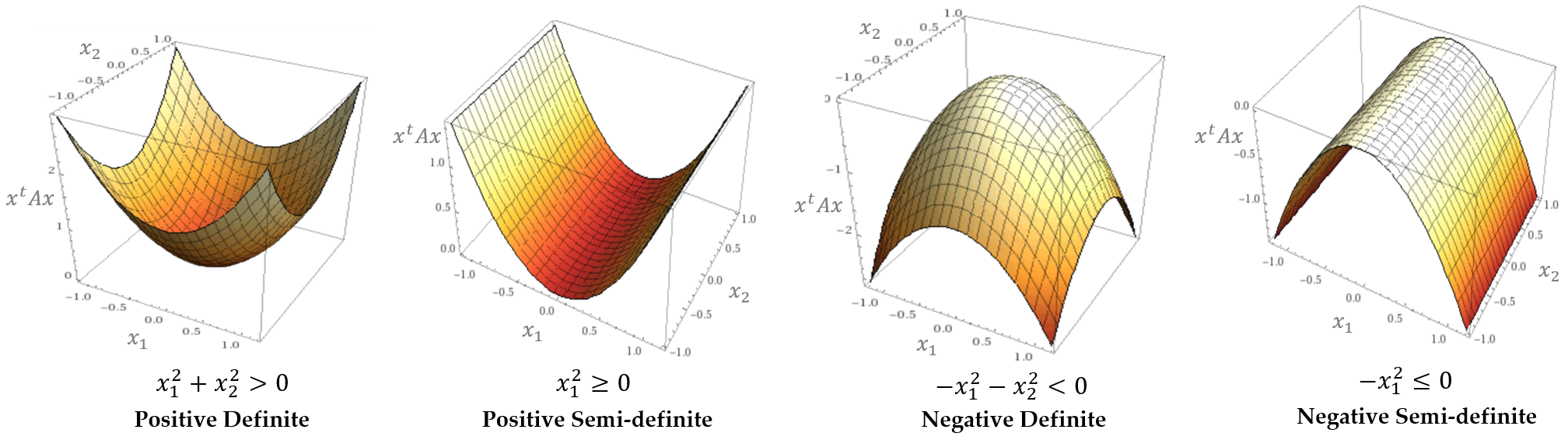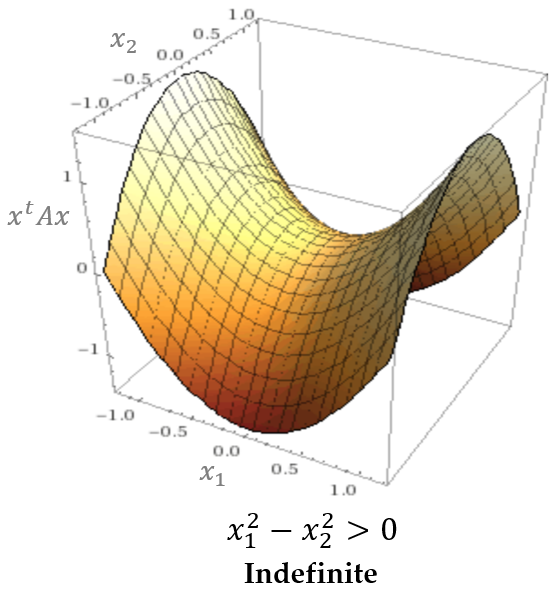# Positive Definiteness

## 1. Definite Matrices

• An $N \times N$ symmetric matrix $A$ is positive definite if and only if for all $N \times 1$ vector $x \not = 0$, $x^t Ax > 0$.
• An $N \times N$ symmetric matrix $A$ is positive semi-definite if and only if for all $N \times 1$ vector $x \not = 0$, $x^t Ax \ge 0$.
• An $N \times N$ symmetric matrix $A$ is negative definite if and only if for all $N \times 1$ vector $x \not = 0$, $x^t Ax < 0$.
• An $N \times N$ symmetric matrix $A$ is negative semi-definite if and only if for all $N \times 1$ vector $x \not = 0$, $x^t Ax \le 0$.
• A matrix that is not positive semi-definite and not negative semi-definite is indefinite.

## 2. What they look like

• Suppose that $A$ is a symmetric $2 \times 2$ matrix and $x \not = 0$ is a $2 \times 1$ vector.
$\begin{equation} A = \left(\begin{array}{cc} a & b \\ b & c \end{array}\right) \implies x^t Ax = \left(\begin{array}{cc} x_1 & x_2 \end{array}\right) \left(\begin{array}{cc} a & b \\ b & c \end{array}\right) \left(\begin{array}{c} x_1 \\ x_2 \end{array}\right) = ax^2_1 + 2bx_1 x_2 + cx^2_2 \end{equation}$• As an example of the positive definiteness, let $a = c = 1$ and $b = 0$. It means $x^t Ax = x^2_1 + x^2_2 > 0$. It looks like a convex function.
• As an example of the positive semi-definiteness, let $a = 1$ and $b = c = 0$. It means $x^t Ax = x^2_1 \ge 0$. It looks like a convex function, but there exists a point such that $x^t Ax = 0$ for $x \not = 0$.
• As an example of the negative definiteness, let $a = c = -1$ and $b = 0$. It means $x^t Ax = -x^2_1 - x^2_2 < 0$. It looks like a concave function.
• As an example of the negative semi-definiteness, let $a = -1$ and $b = c = 0$. It means $x^t Ax = -x^2_1 \le 0$. It looks like a concave function, but there exists a point such that $x^t Ax = 0$ for $x \not = 0$.
• As an example of the indefiniteness, let $a = 1$, $b = 0$, and $c = -1$. It means $x^t Ax = x^2_1 - x^2_2$. It does not look like a shape of the positive and negative definiteness. It has rather a saddle point.## 3. Application: Hessian matrix

• Suppose that $f: \R^n \to \R$ is twice continuously differentiable function and $x$ is a critical point of $f$. According to Taylor’s Theorem,
$\begin{equation} f(x + s) = f(x) + \nabla f(x)^t s + \frac{1}{2}s^t H_f(x + \alpha s)s \end{equation}$

where $s$ is an $N \times 1$ vector, $H_f$ is the Hessian matrix of $f$ and $\alpha \in (0, 1)$. Since $x$ is a critical point, $\nabla f(x) = 0$. Meanwhile, if $H_f$ is positive definite, this property should be also true near $x$, so is $H_f(x + \alpha s)$. It means that $f$ is increasing near $x$. Therefore, $f(x + s) > f(x)$ and $f(x)$ is a local minimum.

• For a critical point $x$, if $H_f$ is positive definite, then $x$ is a minimum of $f$.
• For a critical point $x$, if $H_f$ is negative definite, then $x$ is a maximum of $f$.
• For a critical point $x$, if $H_f$ is indefinite, then $x$ is a saddle point of $f$.
• For a critical point $x$, if $H_f$ is semi-definite, then it requires a higher order differentiation.

## 4. Application: Cholesky factorization

• If $A$ is symmetric and positive (semi-)definite, the Cholesky factorization of $A$ is available, which is arranged so that $LL^t = A$ where $L$ is lower triangular.
• If there exists $L$ such that $LL^t = A$, then for $x \not = 0$, $x^t Ax = x^t LL^t x = (L^t x)^t(L^t x) = \left\| L^t x \right\|^2 \ge 0$. Therefore, $A$ is positive (semi-)definite.
• Here are some examples:
\begin{aligned} A_1 &= \left(\begin{array}{cc} 9 & 6 \\ 6 & 5 \end{array}\right) = \left(\begin{array}{cc} 3 & 0 \\ 2 & 1 \end{array}\right) \left(\begin{array}{cc} 3 & 2 \\ 0 & 1 \end{array}\right) \\ &\implies x^t A_1 x = (3x_1 +2x_2)^2 + x^2_2 > 0: \text{positive definite} \\ \\ A_2 &= \left(\begin{array}{cc} 9 & 6 \\ 6 & 4 \end{array}\right) = \left(\begin{array}{cc} 3 & 0 \\ 2 & 0 \end{array}\right) \left(\begin{array}{cc} 3 & 2 \\ 0 & 0 \end{array}\right) \\ &\implies x^t A_2 x = (3x_1 + 2x_2)^2 \ge 0: \text{positive semi-definite} \\ \\ A_3 &= \left(\begin{array}{cc} 9 & 6 \\ 6 & 3 \end{array}\right) \implies x^t A_3 x = (3x_1 + 2x_2)^2 - x^2_2: \text{indefinite} \\ \\ A_4 &= \left(\begin{array}{cc} 0 & 0 \\ 0 & 4 \end{array}\right) = \left(\begin{array}{cc} 0 & 0 \\ 2 & 0 \end{array}\right) \left(\begin{array}{cc} 0 & 2 \\ 0 & 0 \end{array}\right) = \left(\begin{array}{cc} 0 & 0 \\ 0 & 2 \end{array}\right) \left(\begin{array}{cc} 0 & 0 \\ 0 & 2 \end{array}\right) \\ &\implies x^t A_4 x = 4x_2^2 \ge 0: \text{positive semi-definite} \end{aligned}
• Note that $A_3$ cannot be factorized because it is indefinite. Moreover, the Cholesky factorization can have more than one solution such as $A_4$.

## 5. How to tell if the matrix is positive definite

• All pivots are positive: After Gaussian elimination, pivots are the first non-zero element in each row of a matrix. If all pivots of a matrix are positive, then it is positive definite.
\begin{aligned} A &= \left(\begin{array}{cc} 1 & 2 \\ 2 & 1 \end{array}\right) \implies \left(\begin{array}{cc} 1 & 2 \\ 0 & -3 \end{array}\right) \end{aligned}

After Gaussian elimination, $A$ has the negative pivot, so it is not positive definite.

• All the determinants of upper-left submatrices are positive:
\begin{aligned} A &= \left(\begin{array}{ccc} 2 & -1 & 0 \\ -1 & 2 & -1 \\ 0 & -1 & 2 \end{array}\right) \implies \left\vert\begin{array}{c} 2 \end{array}\right\vert = 2, \left\vert\begin{array}{cc} 2 & -1 \\ -1 & 2 \end{array}\right\vert = 3, \left\vert\begin{array}{ccc} 2 & -1 & 0 \\ -1 & 2 & -1 \\ 0 & -1 & 2 \end{array}\right\vert = 4 \end{aligned}

So, $A$ is positive definite.

• All the eigenvalues are positive:
\begin{aligned} A &= \left(\begin{array}{ccc} 9 & 0 & -8 \\ 6 & -5 & -2 \\ -9 & 3 & 3 \end{array}\right) \implies \lambda_1 = 14.48, \lambda_2 = -5.90, \lambda_3 = -1.57 \end{aligned}

Since there are negative eigenvalues, $A$ is not positive definite.

• By definition: For an $N \times N$ matrix $M$, $M^t M$ is positive (semi-)definite.
\begin{aligned} x^t M^t Mx = (Mx)^t (Mx) = \left\| Mx \right\|^2 \ge 0 \end{aligned}

If $Mx \not = 0$ for all $x \not = 0$, $M^t M$ is positive definite.

## Reference

 Michael T. Heath, Scientific Computing: An Introductory Survey. 2nd Edition, McGraw-Hill Higher Education.